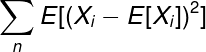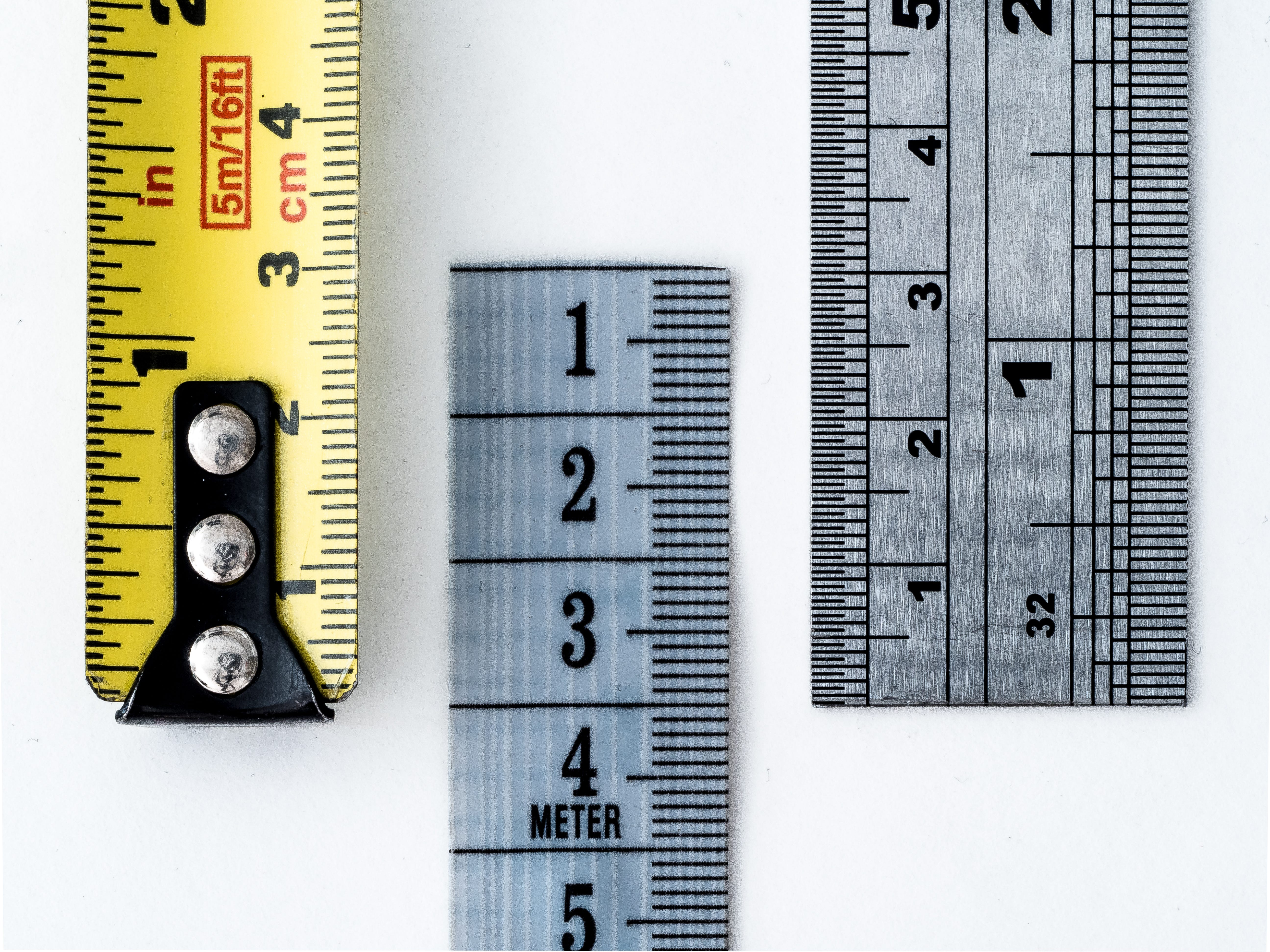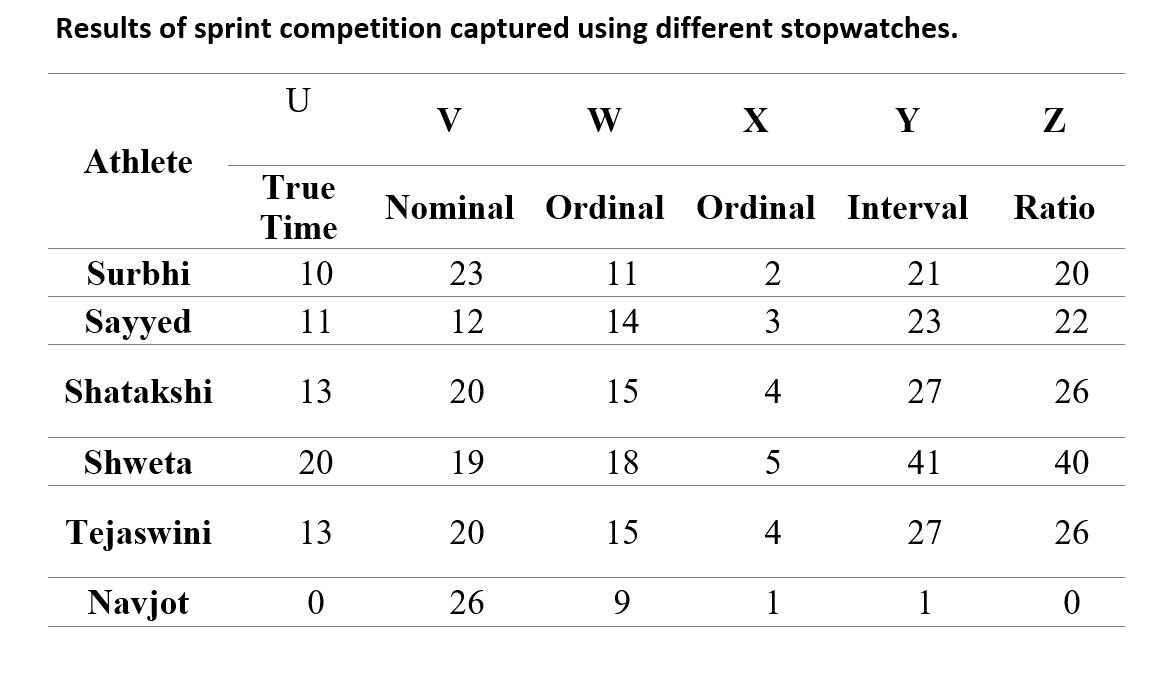1594330800

# How to measure the variance of a statistical model

This article assumes that you understand and know how to build regression or classification models.

The error of any statistical model is composed of three parts — bias, variance and noise. In layman’s terms, bias is the inverse of the accuracy of predictions. And variance refers to the degree to which the predictions are spread out. Noise, on the other hand, is random fluctuation that cannot be expressed systematically.

However, the above definitions are vague and we need to inspect them from a mathematical perspective. In this article, I will stress on variance — the more perplexing demon that haunts our models.

# A short note on Variance

When we allow our models the flexibility to uselessly learn complex relationships over the training data, it loses the ability to generalize. Most of the times, this flexibility is provided through features i.e when the data has a large number of features (sometimes more than the number of observations). It could also be due to a complex neural network architecture or an excessively small training dataset.

What results from this is a model which also learns the noise in the training data; consequently, when we try to make predictions on unseen data, the model misfires.

_Variance is also responsible for the differences in predictions on the same observation in different “realizations” of the model. _We will use this point later to find an exact value for the variance.

# Mathematical explanation

Let Xᵢ be the population of predictions made by model M on observation i. If we take a sample of size n values, the variance will be:Now that was something we already knew. However, we need to calculate the variance of the whole population (which is equivalent to the variance of the statistical model that generated it) and we are not quite there yet. There is one concept we need to understand before that — bootstrap resampling.

Note that this formula of variance assumes that the outcome of the model is a continuous variable — this happens in regression. In the case of classification, the outcome is 0/1 and thus, we would have to measure the variance differently. You can find the explanation to that in this paper.

## Bootstrap Resampling

Often we don’t have access to an entire population to be able to calculate a statistic such as variance or mean. In such cases, we make use of bootstrap sub-samples.

The principle of bootstrapping suggests that if we take a large number of sub-samples of size n with replacement from a sample of size n, then it is an approximation of taking those samples from the original population.

We find the sample statistic on each of these sub-samples and take their mean to estimate the statistic with respect to the population. The number of sub-samples we take is only limited by time and space constraints; however, the more you take, the more accurate will be your result.

## Realizations of a model

Let _M _be our statistical model. A realization of M is a mapping from input to output. When we train M on a particular input, we obtain a specific realization of the model. We can obtain more realizations by training the model on sub-samples from the input data.

#machine-learning #bias-variance #variance-analysis #variance #sensitivity-analysis #data analysis

## Buddha Community1594330800

## How to measure the variance of a statistical model

This article assumes that you understand and know how to build regression or classification models.

The error of any statistical model is composed of three parts — bias, variance and noise. In layman’s terms, bias is the inverse of the accuracy of predictions. And variance refers to the degree to which the predictions are spread out. Noise, on the other hand, is random fluctuation that cannot be expressed systematically.

However, the above definitions are vague and we need to inspect them from a mathematical perspective. In this article, I will stress on variance — the more perplexing demon that haunts our models.

# A short note on Variance

When we allow our models the flexibility to uselessly learn complex relationships over the training data, it loses the ability to generalize. Most of the times, this flexibility is provided through features i.e when the data has a large number of features (sometimes more than the number of observations). It could also be due to a complex neural network architecture or an excessively small training dataset.

What results from this is a model which also learns the noise in the training data; consequently, when we try to make predictions on unseen data, the model misfires.

_Variance is also responsible for the differences in predictions on the same observation in different “realizations” of the model. _We will use this point later to find an exact value for the variance.

# Mathematical explanation

Let Xᵢ be the population of predictions made by model M on observation i. If we take a sample of size n values, the variance will be:Now that was something we already knew. However, we need to calculate the variance of the whole population (which is equivalent to the variance of the statistical model that generated it) and we are not quite there yet. There is one concept we need to understand before that — bootstrap resampling.

Note that this formula of variance assumes that the outcome of the model is a continuous variable — this happens in regression. In the case of classification, the outcome is 0/1 and thus, we would have to measure the variance differently. You can find the explanation to that in this paper.

## Bootstrap Resampling

Often we don’t have access to an entire population to be able to calculate a statistic such as variance or mean. In such cases, we make use of bootstrap sub-samples.

The principle of bootstrapping suggests that if we take a large number of sub-samples of size n with replacement from a sample of size n, then it is an approximation of taking those samples from the original population.

We find the sample statistic on each of these sub-samples and take their mean to estimate the statistic with respect to the population. The number of sub-samples we take is only limited by time and space constraints; however, the more you take, the more accurate will be your result.

## Realizations of a model

Let _M _be our statistical model. A realization of M is a mapping from input to output. When we train M on a particular input, we obtain a specific realization of the model. We can obtain more realizations by training the model on sub-samples from the input data.

#machine-learning #bias-variance #variance-analysis #variance #sensitivity-analysis #data analysis1593564878

## Levels of MeasurementsPhoto by William Warby on Unsplash

Measurement is the process of assigning numbers to quantities (variables). The process is so familiar that perhaps we often overlook its fundamental characteristics. A single measure of some attribute (for example, weight) of sample is called statistic. These attributes have inherent properties too that are similar to numbers that we assign to them during measurement. When we assign numbers to attributes (i.e., during measurement), we can do so poorly, in which case the properties of the numbers to not correspond to the properties of the attributes. In such a case, we achieve only a “low level of measurement” (in other words, low accuracy). Remember that in the earlier module we have seen that the term accuracy refers to the absolute difference between measurement and real value. On the other hand, if the properties of our assigned numbers correspond properly to those of the assigned attributes, we achieve a high level of measurement (that is, high accuracy).

American statistician Stanley Smith Stevens is credited with introducing various levels of measurements. Stevens (1946) said: “All measurements in science are conducted using four different types of scales nominal, ordinal, interval and ratio”. These levels are arranged in ascending order of increasing accuracy. That is, nominal level is lowest in accuracy, while ratio level is highest in accuracy. For the ensuing discussion, the following example is used. Six athletes try out for a sprinter’s position in CUPB Biologists’ Race. They all run a 100-meter dash, and are timed by several coaches each using a different stopwatch (U through Z). Only the stopwatch U captures the true time, stopwatches V through Z are erroneous, but at different levels of measurement. Readings obtained after the sprint is given in Table.# Nominal level of measurement

Nominal scale captures only equivalence (same or different) and set membership. These sets are commonly called categories, or labels. Consider the results of sprint competition, Table 1. Watch V is virtually useless, but it has captured a basic property of the running times. Namely, two values given by the watch are the same if and only if two actual times are the same. For example, participants Shatakshi and Tejaswini took same time in the race (13s), and as per the readings of stopwatch V, this basic property remains same (20s each). By looking at the results from stopwatch V, it is cogent to conclude that ‘Shatakshi and Tejaswini took same time in the race’. This attribute is called equivalency. We can conclude that watch V has achieved only a nominal level of measurement. Variables assessed on a nominal scale are called categorical variables. Examples include first names, gender, race, religion, nationality, taxonomic ranks, parts of speech, expired vs non expired goods, patient vs. healthy, rock types etc. Correlating two nominal categories is very difficult, because any relationships that occur are usually deemed to be spurious, and thus unimportant. For example, trying to figure out how many people from Assam have first names starting with the letter ‘A’ would be a fairly arbitrary, random exercise.

# Ordinal level of measurement

Ordinal scale captures rank-ordering attribute, in addition to all attributes captured by nominal level. Consider the results of sprint competition, Table 1. Ascending order of time taken by the participants as revealed by the true time are (respective ranks in parentheses): Navjot (1), Surbhi (2), Sayyed (3), Shatakshi and Tejaswini (4 each), and Shweta (5). Besides capturing the same-difference property of nominal level, stopwatches W and X have captured the correct ordering of race outcome. We say that the stopwatches W and X have achieved an ordinal level of measurement. Rank-ordering data simply puts the data on an ordinal scale. Examples at this level of measurement include IQ Scores, Academic Scores (marks), Percentiles and so on. Rank ordering (ordinal measurement) is possible with a number of subjective measurement surveys. For example, a questionnaire survey for the public perception of evolution in India included the participants to choose an appropriate response ‘completely agree’, ‘mostly agree’, ‘mostly disagree’, ‘completely disagree’ when measuring their agreement to the statement “men evolved from earlier animals”.

#measurement #data-analysis #data #statistical-analysis #statistics #data analysis1604128560

## An introduction to surrogate modeling, Part III: beyond basics

In part I of this series, we’ve introduced the fundamental concepts of surrogate modeling. In part II, we’ve seen surrogate modeling in action through a case study that presented the full analysis pipeline.

To recap, the surrogate modeling technique trains a cheap yet accurate statistical model to serve as the surrogate for the computationally expensive simulations, thus significantly improving the efficiency of the product design and analyses.

In part III, we will briefly discuss the following three trends emerged in surrogate modeling research and application:

• Gradient-enhanced surrogate modeling: incorporate the gradients at the training samples to improve model accuracy;
• Multi-fidelity surrogate modeling: assimilate training data with various fidelities to achieve higher training efficiency;
• Active learning: train surrogate models intelligently by actively select the next training data.

### 1.1 Basic idea

Gradients are defined as the sensitivity of the output with respect to the inputs. Thanks to rapid developments in techniques like adjoint method and automatic diﬀerentiation, it is now common for engineering simulation code to not only compute the output f(x) given the input vector x, but also compute the gradients ∂_f_(x)/∂**_x _**at the same time with negligible costs.

Consequently, we can expand our training data pairs (xf(x)) to training data triples (xf(x), ∂_f_(x)/∂**x). By leveraging the additional gradient information, the trained surrogate model could reach a higher accuracy compared with the model trained only on (xf(x**)), given that both models use the same number of training data points.

We can also state the benefits of including the gradients in an equivalent way: it allows reducing the number of data points to achieve a given accuracy. This is a desired feature in practice. Recall that generating each training data point requires running the expensive simulation code one time. If we can cut down the total number of training data points, we can train the surrogate model with a smaller computational budget, therefore improving the training efficiency.

#statistics #data-science #modeling #surrogate-modeling #machine-learning1617331277

## Workshop Alert! Deep Learning Model Deployment & Management

The Association of Data Scientists (AdaSci), the premier global professional body of data science and ML practitioners, has announced a hands-on workshop on deep learning model deployment on February 6, Saturday.

Over the last few years, the applications of deep learning models have increased exponentially, with use cases ranging from automated driving, fraud detection, healthcare, voice assistants, machine translation and text generation.

Typically, when data scientists start machine learning model development, they mostly focus on the algorithms to use, feature engineering process, and hyperparameters to make the model more accurate. However, model deployment is the most critical step in the machine learning pipeline. As a matter of fact, models can only be beneficial to a business if deployed and managed correctly. Model deployment or management is probably the most under discussed topic.

In this workshop, the attendees get to learn about ML lifecycle, from gathering data to the deployment of models. Researchers and data scientists can build a pipeline to log and deploy machine learning models. Alongside, they will be able to learn about the challenges associated with machine learning models in production and handling different toolkits to track and monitor these models once deployed.

#hands on deep learning #machine learning model deployment #machine learning models #model deployment #model deployment workshop1618348140

## All Deep Learning Is Statistical Model Building

Deep learning is often used to make predictions for data driven analysis. But what are the meanings of these predictions?
This post explains how neural networks used in deep learning provide the parameters of a statistical model describing the probability of the occurrence of events.

#statistics #machine-learning #deep-learning #modelling #inference